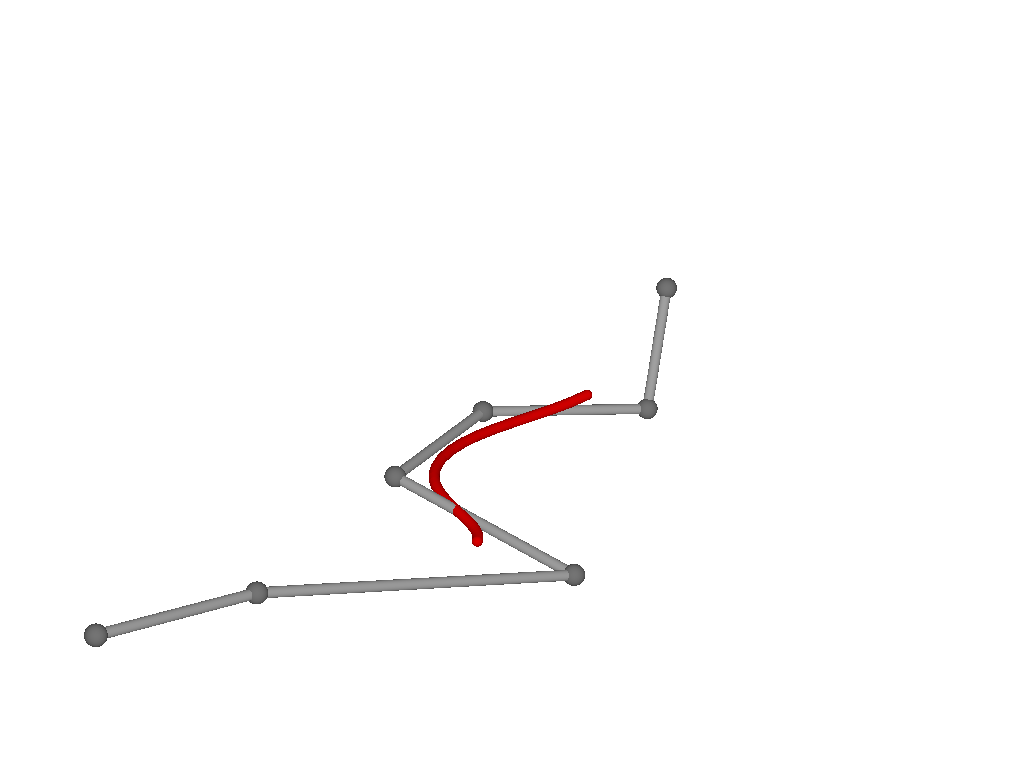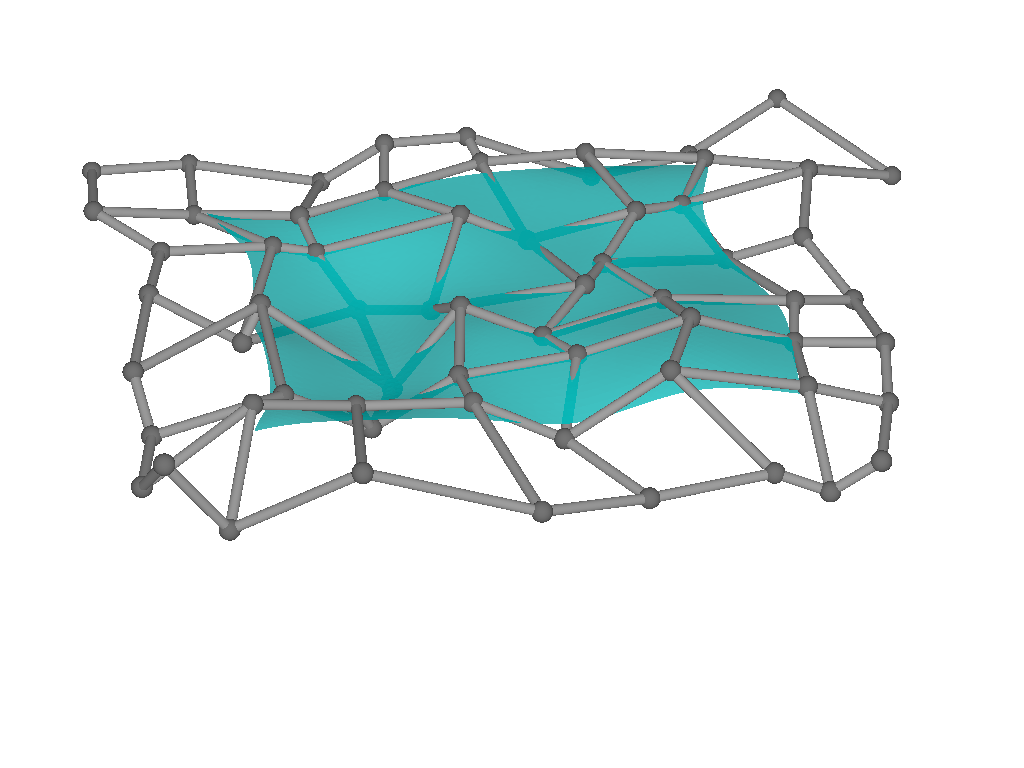# BasicBSplineExporter.jl

BasicBSplineExporter.jl supports export BasicBSpline.BSplineManifold{Dim,Deg,<:StaticVector} to:

• PNG image (.png)
• SVG image (.png)
• POV-Ray mesh (.inc)

## Installation

] add https://github.com/hyrodium/BasicBSplineExporter.jl

## First example

using BasicBSpline
using BasicBSplineExporter
using StaticArrays

p = 2 # degree of polynomial
k1 = KnotVector(1:8) # knot vector
k2 = KnotVector(rand(7))+(p+1)*KnotVector()
P1 = BSplineSpace{p}(k1) # B-spline space
P2 = BSplineSpace{p}(k2)
n1 = dim(P1) # dimension of B-spline space
n2 = dim(P2)
a = [SVector(2i-6.5+rand(),1.5j-6.5+rand()) for i in 1:dim(P1), j in 1:dim(P2)] # random generated control points
M = BSplineManifold(a,(P1,P2)) # Define B-spline manifold
save_png("BasicBSplineExporter_2dim.png", M) # save image## Other examples

Here are some images rendared with POV-Ray.See BasicBSplineExporter.jl/test for more examples.